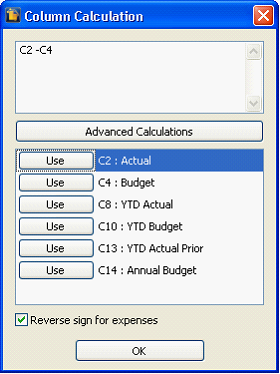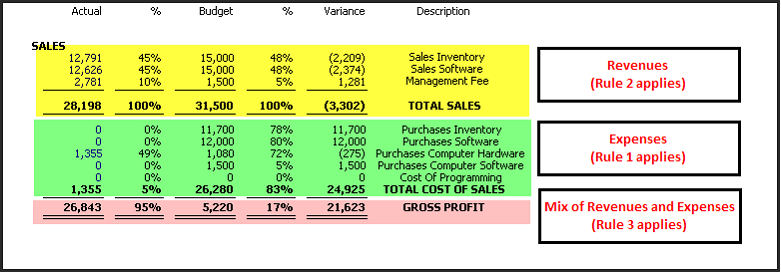﻿ Reverse Sign for Expenses

# Reverse Sign for Expenses

Reverse sign for expenses checkbox on the Column Calculation form will reverse the sign of the total calculation of rows containing Expense accounts.Here are the rules that must be considered when using the reverse sign for expense option:

Rule 1: If all the accounts are Expenses, the following variance applies:

 a) Spent more than the budget, the variance should show negative (unfavorable).
 b) Spent less than the budget, the variable should show positive (favorable).

Rule 2: If all the accounts are Revenues, the following applies:

 a) Earned more than the budget, the variance should show positive (favorable).
 b) Earned less than the budget, the variance should show negative (unfavorable).

Rule 3: Mix of Revenues, Expenses, and/or other account types, the following applies:

 a) Recorded less than the budget, the variance should show negative (unfavorable).
 b) Recorded more than the budget, the variance should show positive (favorable).

Calculation for this report is Actual - Budget = Variance. Notice the amounts for the row where all accounts are expenses, the sign of the Variance amounts are being reversed.